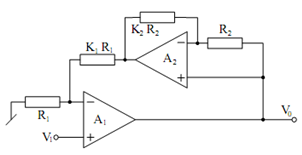## Active-compensated Non-inverting Amplifier Assignment Help

Assignment Help: >> Effect of Op-amp Pole - Active-compensated Non-inverting Amplifier

Active-compensated Non-inverting Amplifier:

In the recent past, there has been considerable research attempt in devising techniques for actively compensating for these effects (for instance see the references cited at the end of the section). The basic idea in all these works has been to alter known op-amp building blocks (using additional op-amps) in such a way that the modified circuits have decreased magnitude and phase errors as compared to the original ones. An exemplary compensated non-inverting amplifier is illustrated in Figure for which an analysis similar to the above yields

v0  / v1= (k1 + 1) { 1 + s τ2/ (1+   s τ1 + s2τ1 τ2 )]

where

τ1 =     K1  + 1 /  ωti , i = 1, 2Figure: Active-compensated Non-inverting Amplifier

From Eq. the magnitude and phase errors (for τ1 = τ2 = τ, ω τ < < 1) are found to be

γ≅ ω2 τ

and

φ≅ - ω3 τ3

Figure. As a result, the compensated non-inverting amplifier of Figure would operate satisfactorily over frequency ranges much higher (typically up to various hundred kHz) than the uncompensated amplifier of Figure. From the circuit of Figure, a compensated inverting amplifier is achievable by grounding the non-inverting inverting input terminal of A1 ungrounding the resistor R1 and applying the input at the node therefore created. Also a compensated buffer is achievable from the circuit of Figure by shorting resistors k1 R1 and k2 R2 and deleting R1 and R2.

Even though we have discussed only one exemplary circuit, a great number of two op-amp and three op-amp based compensated VCVS structures have been evolved up till now. For further information the interested reader is referred to the references cited at the end of this section. Also, the details of similar active compensation techniques evolved for developing the high frequency performance of other useful active circuit building blocks such as integrators, weighted-summers, VCCS, etc. can be found in the references quoted at the end. Therefore, a simple way of developing the high frequency performance of op-amp based building blocks therein by their active compensated versions. The application of this concept in extending the operational frequency range of op-amp RC filters and sinusoidal oscillators is well established now. Details about the improvement of the high frequency performance of well known filters, and the classical Wien bridge oscillator can be found in cited references.